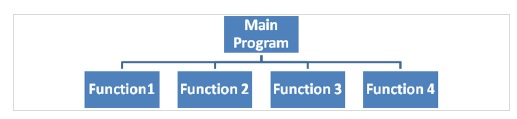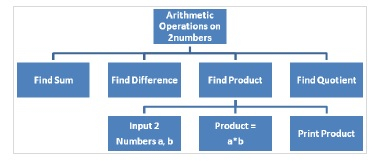# Explain monolithic and modular programming in C language

CServer Side ProgrammingProgramming

The difference between monolithic programming and modular programming along with the advantages and disadvantage are explained below in detail.

## Monolithic programming

If, we write an entire program in a single function that is in main function then, you call it as a monolithic type of programming. But, it is not a good style of writing entire logic in a single function.

The disadvantages of monolithic programming include −

• Program seems to be very large and complex.
• Debugging, testing and maintenance of a program is very difficult.

## Modular Programming

If the program is divided into number of functional parts, then we use to call it as modular programming.

If the main program is divided into sub programs, then we can independently code each sub module later combine into single unit. This type of individual modules is termed as functions.

The advantages of modular programming include −

• It is easy to understand the program.
• Debugging and maintenance becomes easy.
• Saves programmers or user’s time.
• Reuse the code where ever necessary.

Example of modular programming

An example of modular programming is given below −The splitting of a problem into its related sub problems is analogous to the process of refining an algorithm.

Performing arithmetic operations on two numbers

The steps are given below −

• Find sum
• Find difference
• Find product
• Find quotient

Refined algorithm for third step

The refined algorithm to find product of two numbers is as follows −

• take 2 numbers a, b
• product, c = a * b
• print product

## Structure chart

The structure chart is explained below −